# qqconf: An R Package for Putting Testing Bands on QQ and PP Plots

#### 2023-04-14

The qqconf package extends base graphics capabilities to put simultaneous testing bands on Quantile-Quantile (QQ) and Probability-Probability (PP) plots. We support plots for any distribution with a Cumulative Distribution Function (CDF) implemented in R. For the creation of simultaneous testing bands, we support the Kolmogorov-Smirnov (Kolmogorov 1941; Smirnov 1944) method as well as what we refer to as the Equal Local Levels (ELL) method, which conducts an $$\eta$$ level test on each order statistic of the sample supplied such that the global testing bands have some desired $$\alpha$$ Type I error rate. For more information on the computation and properties of ELL, please see our section about ELL Testing Bounds Generation and our paper.

## Installation

Our package is available on CRAN and can be installed by running:

install.packages("qqconf")

## Usage

### Plotting

The package contains two functions for creating plots, qq_conf_plot and pp_conf_plot to create QQ plots and PP plots, respectively. These two functions have identical interfaces, with the exception that qq_conf_plot requires the input of a quantile function (e.g. qnorm) and pp_conf_plot requires the input of a distribution function (e.g. pnorm). Thus, any information below about parameters that can be fed to the qq_conf_plot function also apply to pp_conf_plot, and vice versa.

#### Basics / Parameter Estimation

First, we load in qqconf

require(qqconf)

Then, we generate data from a standard normal distribution:

set.seed(0)
sample <- rnorm(n = 100, mean = 0, sd = 1)

Now, if we did not know the distribution that our sample came from but instead wanted to test if it was sampled i.i.d from a $$N(0, 1)$$ distribution, we could create a QQ plot to compare the quantiles of the sample to the theoretical quantiles of a $$N(0, 1)$$ distribution.

To do this, we call qq_conf_plot as follows:

qq_conf_plot(
obs = sample,
distribution = qnorm,
dparams = list(mean = 0, sd = 1)
)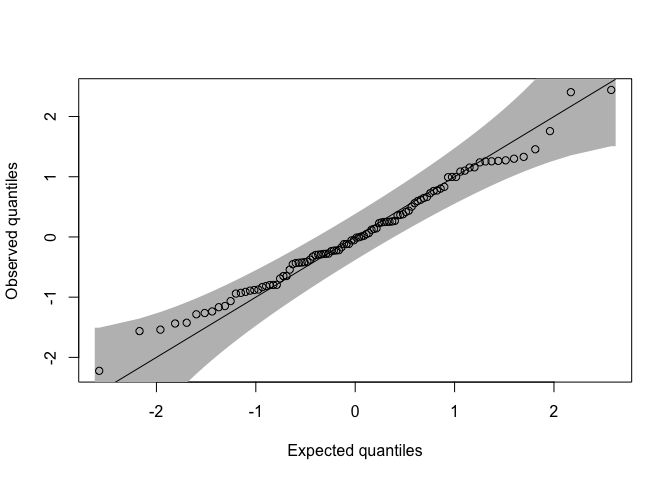By default, this function creates a QQ plot with ELL testing bounds calculated with a Type I error rate of .05. Note here that dparams is not a required parameter. If we were to call qq_conf_plot without specifying dparams, then our code would estimate the mean and variance of the sample assuming it comes from a normal distribution as is specified by the distribution parameter. More generally, for any continuous distribution that is neither normal nor uniform, we use maximum liklihood estimation to estimate all parameters of the distribution. For the uniform distribution, we do not support parameter estimation but instead default to $$U(0, 1)$$ and allow the user to specify a custom max and min. For the normal distribution, because it is so commonly used as the reference distribution in QQ plots, we experimented with different methods of parameter estimation and found that estimating the mean of the distribution as the median of the sample and the standard deviation as $$S_{n}$$ as proposed by Rousseeuw and Croux (Rousseeuw, Crow 1993) had well calibrated Type I error rates. When we used the MLEs for the normal distribution, we found that the testing bounds produced were conservative. Note that the testing bounds produced for other distributions with parameters estimated via MLE will also likely be conservative. For more information on parameter estimation, please see section 2.6 of our paper.

#### Modifying Visual Features

##### General Plot Features

Parameter estimation aside, we have now created a QQ plot that compares our sample to $$N(0, 1)$$. While this plot is informative, there are a number of potential modifications we may want to make to this plot for ease of viewing. First, because the top and the bottom of the confidence bands are cut off in this plot, we may want to expand the y-axis limits. We do this by simply adding a ylim argument to the function call:

qq_conf_plot(
obs = sample,
distribution = qnorm,
dparams = list(mean = 0, sd = 1),
ylim = c(-4, 4)
)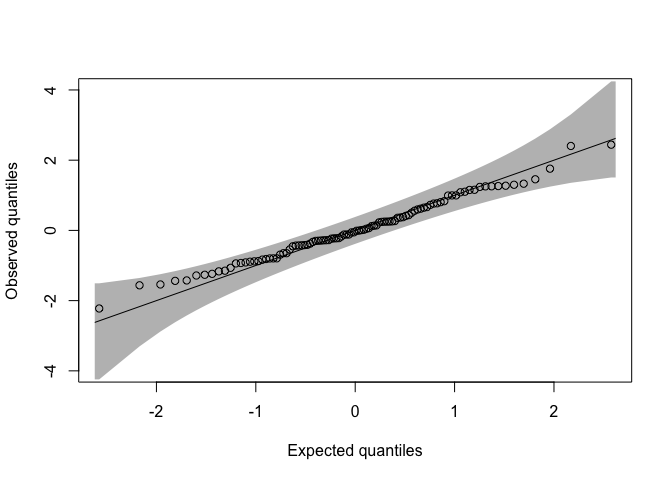In fact, because qq_conf_plot takes ... as an argument, any other parameter normally passed to the plot function in base can be passed to qq_conf_plot. If, for instance, we wanted to modify the axis labels, we could write:

qq_conf_plot(
obs = sample,
distribution = qnorm,
dparams = list(mean = 0, sd = 1),
ylim = c(-4, 4),
)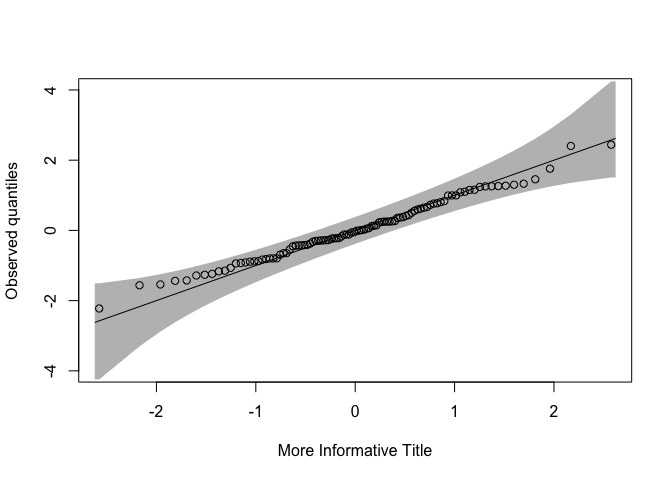However, while the ... argument can be used for more general features of the plot like axis titles, because there are so many separate objects in the plot (points, lines, bands), we’ve added additional arguments so that the user can easily specify the visual features of any element of the plot.

##### Points

If we want to modify the appearance of the points on the plot, we can use the optional points_params argument. This argument is a list that takes any arguments that can be passed to the points function in base. For instance, if we wanted to make the points smaller, we could do the following.

qq_conf_plot(
obs = sample,
distribution = qnorm,
dparams = list(mean = 0, sd = 1),
ylim = c(-4, 4),
points_params = list(cex = .5) # makes points smaller
)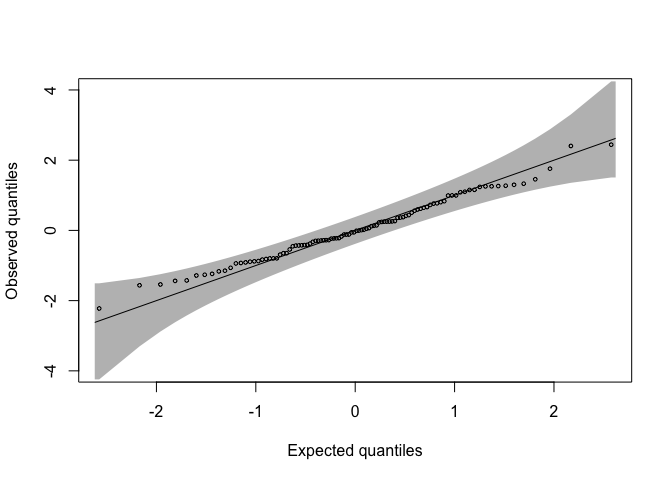For more options, see the documentation of the points function.

##### Expectation Line

If we want to modify the line in the plot that indicates a perfect fit between the reference distribution and the sample, we can use the line_params argument. This list can take any parameters that can be passed to the lines function and will modify the plot accordingly. If, for instance, we wanted to make the line red, we would do the following:

qq_conf_plot(
obs = sample,
distribution = qnorm,
dparams = list(mean = 0, sd = 1),
ylim = c(-4, 4),
line_params = list(col="red") # makes expectation line red
)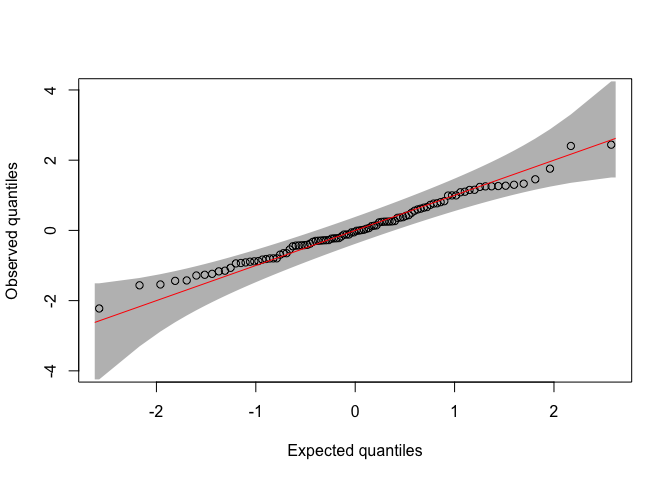Note, that if we don’t want this line to show up on the plot at all, we can pass in type = "n" to line_params.

qq_conf_plot(
obs = sample,
distribution = qnorm,
dparams = list(mean = 0, sd = 1),
ylim = c(-4, 4),
line_params = list(type="n") # removes expectation line
)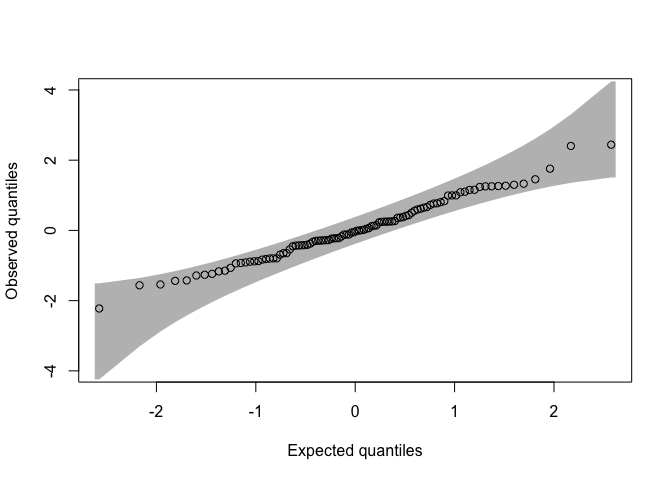Again, for more information see the documentation for the lines function.

##### Testing Bands

By default, qq_conf_plot does not plot pointwise testing bands on the plot. Pointwise testing bands, in contrast to simultaneous testing bands, are anti-conservative, as they ignore the multiple testing problem inherent in QQ plots and simply conduct a test on each order statistic. To add these pointwise bands, one can simply set the plot_pointwise parameter to True in the qq_conf_plot function. To modify their appearance, one can use the pointwise_lines_params parameter. Because this parameter list is simply passed to the lines function, it works exactly the same way as the line_params parameter.

Finally, if we want to modify the appearance of the simultaneous testing bands, we can use the polygon_params parameter. As the name suggests, arguments in this parameter list are passed to the polygon function in base and modify the testing bands accordingly. Note that by default, border is set to NA and col is set to grey. If the user overrides the default option, all defaults of polygon are used unless otherwise specified, which does not set border to NA and col to grey. If, for instance, we wanted to change the color of the shading, we would do the following:

qq_conf_plot(
obs = sample,
distribution = qnorm,
dparams = list(mean = 0, sd = 1),
ylim = c(-4, 4),
polygon_params = list(col = 'powderblue', border = NA) # change shading and keep no border
)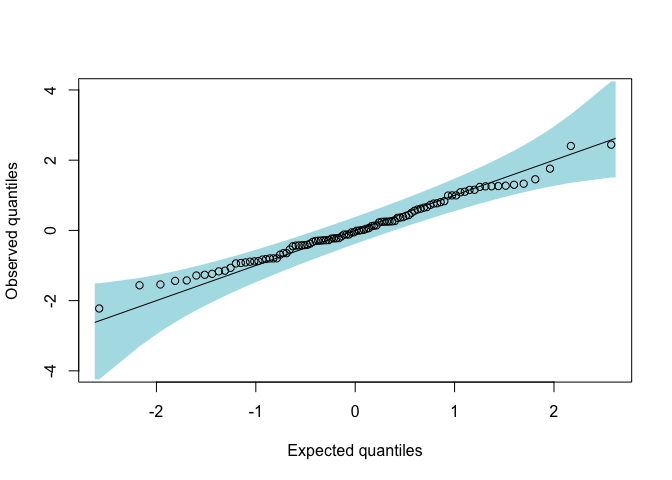#### Scaling and Data Transformations

In addition to general visual parameters to modify the elements of the plot, we’ve also included a few parameters that we found to be useful in particular cases when making a QQ or PP plot. Especially when $$n$$ is large, it can be difficult to see the relevant parts of the plot.

To demonstrate this, we will generate 10,000 points from a $$Beta(1, 1.05)$$ distribution and test this sample against a $$U(0, 1)$$ distribution.

sample <- rbeta(n = 10000, shape1 = 1.0, shape2 = 1.05)

Because a QQ plot and PP plot are equivalent for the uniform distribution, we will switch to PP plots to demonstrate the interface.

##### Differencing

Normally in a PP plot, the expected probabilities are plotted on the x-axis and the theoretical probabilities are plotted on the y-axis.

pp_conf_plot(
obs = sample,
distribution = punif,
points_params = list(cex=.1)
)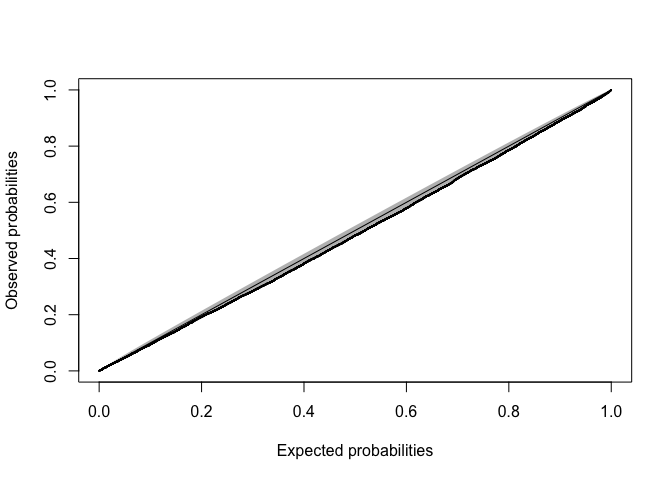However, in this plot it is very difficult to see anything because the individual points are so small and the magnitude of the deviation from the reference distribution is not very large.

Instead, if we plot the expected probabilities on the x-axis and the observed probabilities - the expected probabilities on the y-axis by setting the difference parameter to TRUE, things become much easier to see:

pp_conf_plot(
obs = sample,
distribution = punif,
points_params = list(cex=.1),
difference = TRUE, # Make y-axis differenced
ylim = c(-.0225, .0225)
)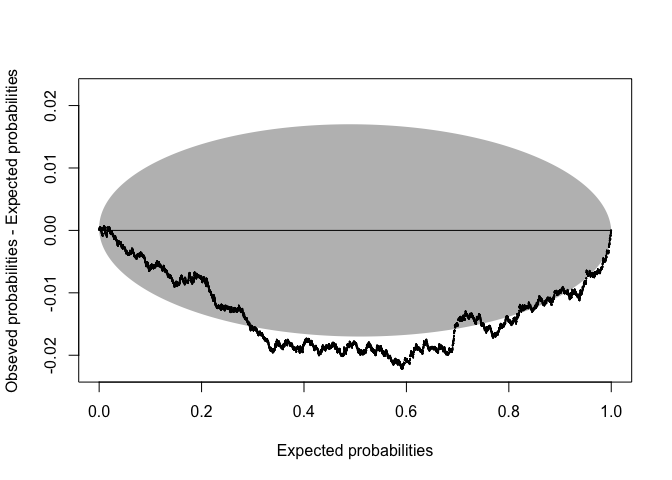##### log Scaling

Sometimes, we are only interested in deviations of the sample from one tail of the reference distribution. For instance, if we have a sample of $$n$$ p-values that we would like to compare to the $$U(0, 1)$$ distribution for the sake of global null hypothesis testing, we’re generally only interested in p-values that are small. In this case, especially with large $$n$$, it can be helpful to transform the probability values onto the $$-log10$$ scale.

To demonstrate this, we will generate 10,000 points from a mixture of a $$Beta(.25, 1)$$ and a $$U(0, 1)$$ and test this sample against a $$U(0, 1)$$ reference distribution.

mix <- distr::UnivarMixingDistribution(
distr::Beta(shape1 = .25, shape2 = 1),
distr::Unif(),
mixCoeff=c(.01, .99)
)

sampler <- distr::r(mix)

sample <- sampler(10000)

Again, if we make a standard PP plot the relevant parts of the plot are very difficult to make out.

pp_conf_plot(
obs = sample,
distribution = punif,
points_params = list(cex=.1)
)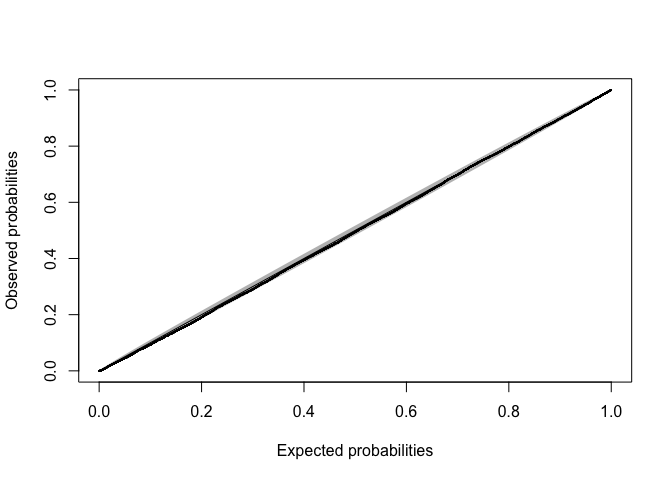Even if we set difference to TRUE, it’s still difficult to see the left tail of the distribution.

pp_conf_plot(
obs = sample,
distribution = punif,
points_params = list(cex=.1),
difference = TRUE
)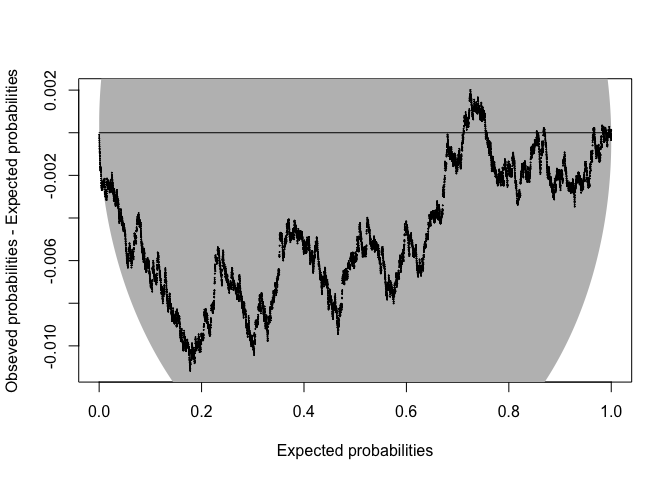However, setting log10 to TRUE makes the plot much easier to see.

pp_conf_plot(
obs = sample,
distribution = punif,
points_params = list(cex=.1),
log10 = TRUE
)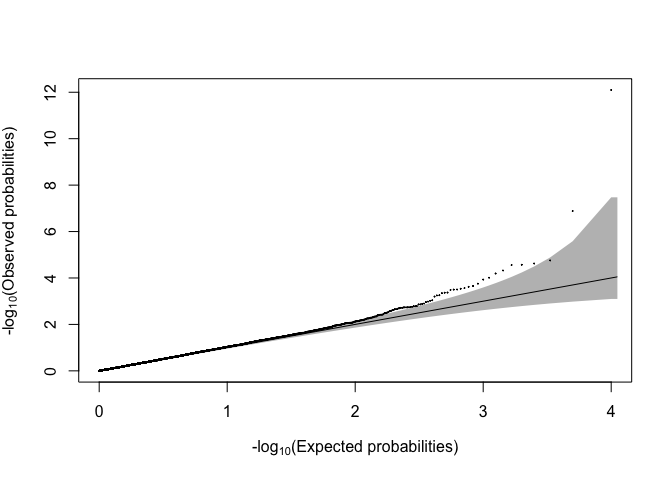Note, that if we want to highlight the right tail of the distribution as opposed to the left tail, we can set log10 to TRUE and the right_tail parameter to TRUE. This results in a plot with the data transformed onto the log10 scale instead of the -log10 scale.

### ELL Testing Bounds Generations

In addition to the plotting interfaces explained above, qqconf provides functions to directly produce testing bounds generated by the equal local levels method. Functions exist for both two-sided and one-sided testing. For information on the generation of testing bounds using equal local levels, please see section 2 of our paper.

#### Two-Sided Testing

Given a desired global Type I error rate $$\alpha$$ and a sample size $$n$$, we can generate two-sided bounds using the get_bounds_two_sided function. Below, we generate such bounds for $$n = 100$$ and $$\alpha = .05$$:

bounds <- get_bounds_two_sided(alpha = .05, n = 100)

In this case, the bounds object is a list with a number of objects (for more information see the documentation), but the lower bounds can be extracted with bounds$lower_bounds and the upper bounds with bounds$upper_bounds.

#### One-Sided Testing

In addition to two-sided testing, qqconf also provides functionality for one-sided testing.

Given a desired global Type I error rate $$\alpha$$ and a sample size $$n$$, we can generate such bounds using the get_bounds_one_sided function. Below, we generate such bounds for $$n = 100$$ and $$\alpha = .05$$:

bounds <- get_bounds_one_sided(alpha = .05, n = 100)

From the bounds object, the upper bounds can be extracted via bounds\$bound. Again, this function returns a list and more information can be extracted from bounds.

## Session info

All plots above were generated with the following session information:

sessionInfo()
#> R version 4.2.2 (2022-10-31)
#> Platform: x86_64-apple-darwin17.0 (64-bit)
#> Running under: macOS Big Sur ... 10.16
#>
#> Matrix products: default
#> BLAS:   /Library/Frameworks/R.framework/Versions/4.2/Resources/lib/libRblas.0.dylib
#> LAPACK: /Library/Frameworks/R.framework/Versions/4.2/Resources/lib/libRlapack.dylib
#>
#> locale:
#>  C/en_US.UTF-8/en_US.UTF-8/C/en_US.UTF-8/en_US.UTF-8
#>
#> attached base packages:
#>  stats     graphics  grDevices utils     datasets  methods   base
#>
#> other attached packages:
#>  qqconf_1.3.2
#>
#> loaded via a namespace (and not attached):
#>   Rcpp_1.0.10      digest_0.6.31    MASS_7.3-58.3    distr_2.9.1
#>   R6_2.5.1         jsonlite_1.8.4   evaluate_0.20    highr_0.10
#>   rlang_1.1.0      cachem_1.0.7     cli_3.6.1        jquerylib_0.1.4
#>  bslib_0.4.2      rmarkdown_2.21   tools_4.2.2      sfsmisc_1.1-14
#>  xfun_0.38        yaml_2.3.7       fastmap_1.1.1    compiler_4.2.2
#>  htmltools_0.5.5  knitr_1.42       startupmsg_0.9.6 sass_0.4.5

1. University of Chicago, ↩︎

2. University of Chicago, ↩︎

3. University of Chicago, ↩︎